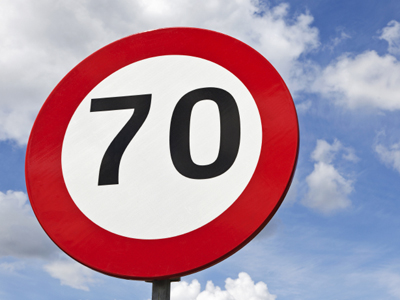10 x 7 = 70.

# 10 Times Table

The ten times table may well be the easiest of them all - but you still need to learn it. It'll come in very useful in everyday life and in your 11-Plus. So, even though you may think it's very simple, practise it all the same.

Multiplication by 10 is what the ten times table is all about. Ten is a very important number because the metric system bases its units on multiples of ten. There are tem millimetres in a centimetre, a hundred centimetres in a metre, a thousand metres in a kilometre - the list goes on. Grams, litres, hectares. They all work by using multiples of ten. Thankfully there is a very simple way to multiply a number by ten - you just add a zero to its end. 7 x 10 = 70. 56 x 10 = 560. 1,267 x 10 = 12,670. What could be easier?

With that in mind, you may be feeling very confident of getting top marks in this quiz - but be careful. If you don't concentrate then you might click on the wrong answer by mistake. Take your time and consider your answer before you make your choice. Good luck!

1.
10 × 12 = ?
1,200
120
121
1.20
There are 120 hours in 5 days
2.
10 × 11 = ?
110
111
100
0.1
Add a zero to the end of 11 to make 110
3.
10 × 7 = ?
7
700
0.7
70
Add a zero after the 7 to multiply it by 10
4.
10 × 5 = ?
5
500
50
0.5
50 is the same as one half of 10 x 10
5.
10 × 9 = ?
9
900
0.9
90
90 is 10 less than 100, which is 10 x 10
6.
10 × 8 = ?
80
0.8
800
8
Just add a 0 after the 8 and you get the answer 80
7.
10 × 4 = ?
40
400
4
0.4
4 x 10 is the same as 10 + 10 + 10 + 10
8.
10 × 2 = ?
200
0.2
20
2
There are 20 sectors on a dartboard
9.
10 × 3 = ?
300
3
30
0.3
10 x 3 is found by adding a 0 to the end of 3
10.
10 × 6 = ?
600
60
6
0.6
There are 60 seconds in a minute and 60 minutes in an hour
Author:  Frank Evans